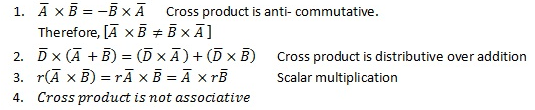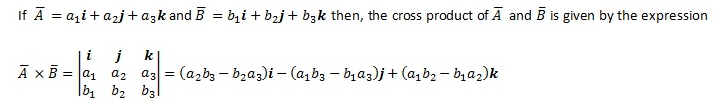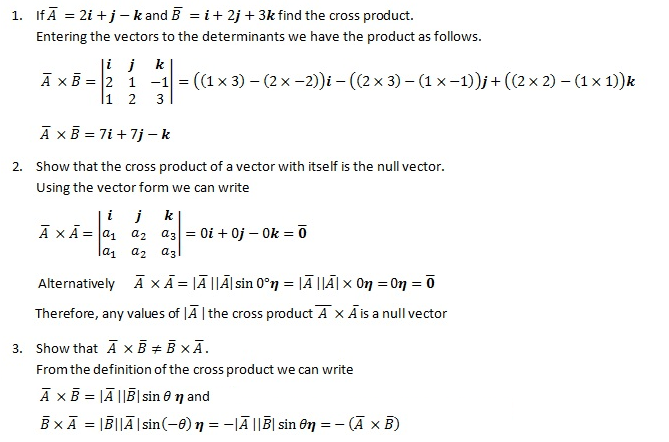# How to Find the Cross Product

As cross product or the vector product is a binary operation between two vectors in three-dimensional vector space, knowing how to find the cross product is useful. The cross product of two vector results in another vector perpendicular to the plane containing first two vectors. In general, cross product or vector product is symbolized by multiplication sign, but the mathematical operation is more advanced than simple algebraic multiplication.

The cross product of vectors and is denoted as and produces another vector , which is perpendicular to both and .where θ is the angle measured from   to and η is the unit vector in the direction perpendicular to the plane containing both and .

Geometrically, the magnitude of the cross product of two vectors is equal to the area of a parallelogram with and as adjacent sides. Vectors , and for a right-handed system as follows:The cross product has the following algebraic properties.Following Results also hold for cross product.

## How to find the Cross Product

Vectors are often given in terms of components within a coordinate system. When given in such form, it is convenient to use determinants to calculate the cross product.Above result is for the Cartesian coordinates.

## How to find the cross product – ExamplesHence, cross product is not commutative.